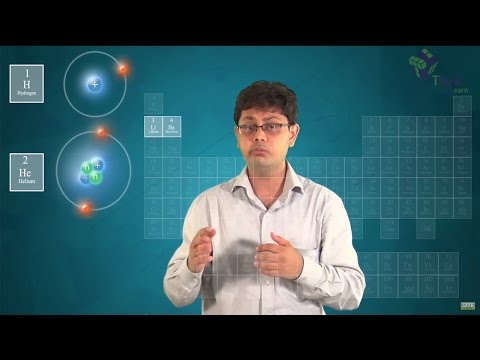# Isotopes of ElementsElements are fundamental substances which have same type of atoms. All the elements are electrically neutral that is they can neither be positive nor can they be negative. Let’s see how elements are made. Take one proton that is positively charged and hook it up with one electron that is negatively charged. Combining both of them we find an element that is Hydrogen. Now if we take two protons and try putting them together to make an element, then it is not possible as both of them have same charge. So they will repel each other. To make them stable we will add a neutral particle i.e. neutron.

But now the element is positively charged, and we need the element to be neutral so we will add two electrons to the element. Thus, the Electrons and Protons will bind together with a stronger electrostatic force, and we will get a helium atom. So to make elements we have to add electrons, protons and neutrons in it.

In the same way, if we keep on increasing the number of protons and electrons then we get different elements. Like for 3 protons, we get lithium element, 4 protons – Beryllium, 5- Boron, 6- Carbon, 7- Nitrogen, 8- Oxygen, etc. So for one proton the element is hydrogen and for 92 it is uranium. Beyond Uranium, the nuclei become larger and unstable. So we can say that by increasing electrons and protons we get new elements. But what if we increase the number of neutrons?

By increasing the number of neutrons, we can find different forms of the same element that are known as the isotopes of the element. Isotopes of the same element are defined as the elements having the same number of protons but a different number of neutrons. For example: Carbon-12, carbon-13 and carbon-14. Carbon atom having 6 protons and 6 neutrons are carbon 12. When one extra neutron is added in this atom then it is known as carbon 13. It is heavier than C-12 atom. When 2 neutrons are added to carbon-12 atom, then it is known as Carbon-14 atom.

#### Practise This Question

Consider the situation shown in figure. Initially the spring is unstretched when the system is released from rest. Assuming no friction in the pulley, find the maximum elongation of the spring?NCERT Related Articles NCERT Solutions for Class 12 NCERT Solutions for Class 11 NCERT Solutions for Class 10 NCERT Solutions for Class 12 Maths NCERT Solutions for Class 11 Maths NCERT Solutions for Class 10 Maths NCERT Solutions for Class 12 Physics NCERT Solutions for Class 11 Physics NCERT Solutions for Class 10 Science NCERT Solutions for Class 12 Chemistry NCERT Solutions for Class 11 Chemistry NCERT Solutions for Class 6 NCERT Solutions for Class 12 Biology NCERT Solutions for Class 11 Biology NCERT Solutions for Class 6 Maths NCERT Solutions for Class 9 NCERT Solutions for Class 8 NCERT Solutions for Class 6 Science NCERT Solutions for Class 9 Maths NCERT Solutions for Class 8 Maths NCERT Solutions for Class 4 NCERT Solutions for Class 9 Science NCERT Solutions for Class 8 Science NCERT Solutions for Class 4 Maths NCERT Solutions for Class 5 NCERT Solutions for Class 7 NCERT Solutions for Class 7 Maths NCERT Solutions for Class 4 Science NCERT Solutions for Class 5 Maths NCERT Solutions for Class 7 Science NCERT Solutions NCERT Books NCERT Solutions for Class 5 Science